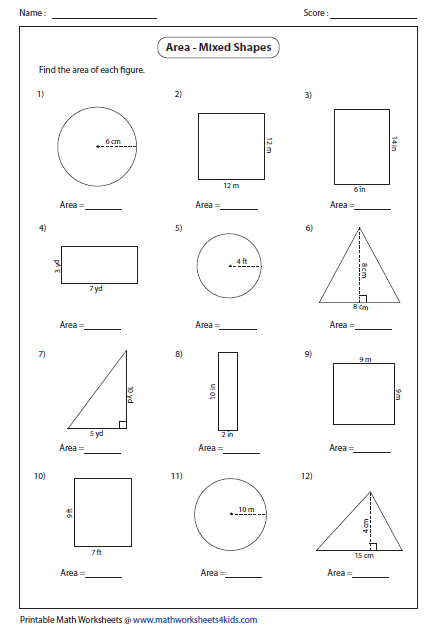# Making geometry worksheets in LaTeX?

I work as a math tutor and I'd like to make geometry worksheets for 7th grade students: These worksheets would include finding the area of various polygons, and finding the volume of various shapes. I would just need some package that can draw the shape for me and allow me to insert various lengths/widths/heights. Is there such a package?

Edit:

Thank you for the warm welcomes. Just to give more details I would be looking to make something like thisIs this possible in the TikZ package?

Here is something to get you started. The configuration assumes that each row of the worksheet will include 3 questions. However, you can easily adapt it if necessary.

As far as the figures are concerned, the only one which seems worth making a little more automatic is the circle because that always follows the same structure. In other cases, the labels etc. vary and, since the shapes are simple, you might just as soon draw them as you wish.

tikzqn is an environment which will automatically number the questions and insert answer spaces.

\documentclass[a4paper]{article}
\usepackage[scale=.85]{geometry}
\usepackage{tikz}
\usetikzlibrary{shapes.geometric}
\newcounter{tikzqn}
\newcounter{tikzrow}
\newenvironment{tikzqn}%
{%
\stepcounter{tikzqn}%
\stepcounter{tikzrow}%
\begin{minipage}[t]{.3\textwidth}
\sffamily\thetikzqn)\par\centering
\begin{tikzpicture}[baseline=(current bounding box.north), font=\sffamily, thick]
}{%
\end{tikzpicture}
\bigskip\vfill\par
\end{minipage}\hfill
\ifnum\value{tikzrow}=3\setcounter{tikzrow}{0}%
\bigskip\par
\foreach \i in {1,2,3}
{\begin{minipage}{.3\textwidth}
\end{minipage}\hfill}%
\fi}
\pagestyle{empty}
\usepackage{cabin}
\begin{document}
\tikzset{
pics/my circle/.style 2 args={
code={
\draw circle (#1);
\draw [fill] (0,0) circle (1pt) -- (#1,0) node [midway, above] {#2};
}
},
}
\noindent
\begin{tikzqn}
\pic {my circle={20mm}{71cm}};
\end{tikzqn}
\begin{tikzqn}
\draw (0,0) -- ++(40mm,0) node [midway, below] {37cm} -- ++(0,20mm) node [midway, right, anchor=north, sloped] {21cm} -| cycle;
\end{tikzqn}
\begin{tikzqn}
\draw (0,0) coordinate (a) -- ++(20mm,0) node [midway, below] {10cm} -- ++(-10mm,25mm) coordinate (c) -- cycle;
\draw [dashed] (a -| c) -- (c) node [pos=.35, right, anchor=south, sloped] {8cm};
\draw (a -| c) ++ (0,5pt) -| ++(5pt,-5pt);
\end{tikzqn}

\begin{tikzqn}
\draw (0,0) -- ++(0,40mm) node [midway, right, anchor=south, sloped] {3m} -| ++(10mm,-40mm) -- cycle node [midway, below, anchor=north] {75cm};
\end{tikzqn}
\begin{tikzqn}
\pic {my circle={15mm}{4cm}};
\end{tikzqn}
\begin{tikzqn}
\draw (0,0) -| ++(20mm,25mm) coordinate [midway] (b) coordinate (c) -- cycle;
\path (0,0) -- (b) node [midway, below, anchor=north] {11cm} -- (c) node [midway, right, anchor=north, sloped] {17cm};
\draw (b) rectangle ++(-5pt,5pt);
\end{tikzqn}

\begin{tikzqn}
\node (dia) [draw, shape=diamond, minimum size=30mm] {};
\node [rotate=45, anchor=north] at (dia.south east)  {6cm};
\draw (dia.north) ++(-2.5pt,-2.5pt) -- ++(2.5pt,-2.5pt) -- ++(2.5pt,2.5pt);
\draw (dia.south) ++(-2.5pt,2.5pt) -- ++(2.5pt,2.5pt) -- ++(2.5pt,-2.5pt);
\end{tikzqn}
\begin{tikzqn}
\node (sc) [draw, shape=semicircle, minimum size=25mm, label=below:46mm] {};
\draw [dashed] (sc.south) -- (sc.north) node [midway, left, anchor=south, sloped] {23mm};
\draw (sc.south) ++(5pt,0) |- ++(-5pt,5pt);
\end{tikzqn}
\begin{tikzqn}
\draw (0,0) -- ++(35mm,0) coordinate (a) node [midway, below, anchor=north] {14cm} arc (-90:90:15mm) coordinate (b) -| cycle;
\draw [dashed] (a -| b) -- (b) node [midway, right, anchor=south, sloped] {5cm};
\draw [dashed] ([yshift=15mm]a) coordinate (c) -- ++(15mm,0) node [midway, below, anchor=north] {2.5cm};
\draw (a) ++(-5pt,0) |- ++(5pt,5pt) (c) ++(5pt,0) |- ++(-5pt,5pt);
\end{tikzqn}

\begin{tikzqn}
\node (tri) [draw, regular polygon, regular polygon sides=3, label=-90:10cm, minimum size=45mm] {};
\path (tri.corner 2) -- (tri.corner 1) node [midway, left, anchor=south, sloped] {10cm};
\path (tri.corner 3) -- (tri.corner 1) node [midway, left, anchor=south, sloped] {10cm};
\end{tikzqn}
\begin{tikzqn}
\node (sq) [draw, regular polygon, regular polygon sides=4, label=-90:8cm, minimum size=40mm] {};
\path (sq.south east) -- (sq.north east) node [midway, right, sloped, anchor=north] {8cm};
\end{tikzqn}
\begin{tikzqn}
\node [draw, regular polygon, regular polygon sides=5, label=-90:7cm, minimum size=35mm] {};
\end{tikzqn}

\end{document}• Thanks for changing the orientation of the vertical lengths' labels. Jul 10, 2015 at 21:58
• @Manuel Why? Or is that an objection?
– cfr
Jul 10, 2015 at 22:01
• I just reread, and it definitely looks like an objection hehe It was a real thanks. Why? Because the other way is just antinatural for my body. Jul 10, 2015 at 22:02
• @Manuel Until you said it, I didn't even realise I'd done it. I think I just did it the way which 'made sense' to me. I don't know if that is because this is a more common orientation or because of my eyes or what.
– cfr
Jul 10, 2015 at 22:05
• It's the most common way because it's the standard in acotation (I think some kind of ISO or something like that). In any case it was the natural way to my body quite before I found out about it in school (I don't know why the hell I was thinking about this kind of things when I was soooo young). Jul 10, 2015 at 22:07